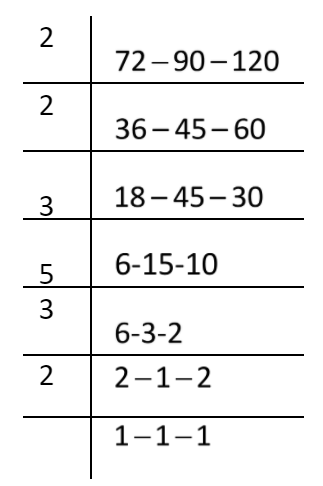QuestionAnswers

# Find the LCM of the following using prime factorization method: $72,90,120.$Hint: LCM is elaborated as Least Common Multiple, which is the lowest common factor among the integers. To find the LCM of the given numbers, which is the lowest common number, which is divisible by all numbers for which we are finding the LCM, the method includes basic factorization of the numbers to find factors that are multiplied together to form a number. If the all given numbers are $0$ for which LCM is being calculated, their LCM will also be $0$. First, find the factors of the number that are the number when multiplied together gives the original number.

To find the lowest common multiple of the numbers, let’s find the factors of the given numbers for which we have to find the LCM of each number $72,90,120.$.
Factors are the integers, which, when multiplied together results in the original number only. First, find the factors of numbers.Now multiply the common multiple with the other factors of the LCM:
$LCM = 2 \times 2 \times 3 \times 5 \times 3 \times 2 \\ = 360 \\$
Hence, the LCM of the numbers $72,90,120$ is 360.

Note: LCM of given numbers is exactly divisible by each of the numbers. During the LCM calculation, students must know the tables of various numbers, and they have to perform the operations step by step. Lastly, they have to multiply all the numbers by which they are dividing the given set of numbers. The least common multiple, lowest common multiple, or smallest common multiple of two integers a and b, usually denoted by LCM (a, b), is the smallest positive integer that is divisible by both a and b.
Prime Factorization Of HCF And LCMApplication of LCM and HCFTo Find the Weight of a Given Body Using Parallelogram Law of VectorsLCM FormulaSquare Root Prime FactorizationTo Determine the Mass of Two Different Objects Using a Beam BalanceTo Measure the Volume of an Irregular Lamina Using Screw GaugeRelation Between the Length of a Given Wire and Tension for Constant Frequency Using SonometerThe Idea of TimePrime NumbersCBSE Class 8 Science Reaching The Age of Adolescence WorksheetsImportant Questions for CBSE Class 8 English Honeydew Chapter 3 - Glimpses of the PastImportant Questions for CBSE Class 8 Science Chapter 10 - Reaching The Age of AdolescenceImportant Questions for CBSE Class 11 Biology Chapter 8 - Cell The Unit of LifeCBSE Class 8 Science Stars and The Solar System WorksheetsImportant Questions for CBSE Class 8 Social Science Our Past 3 Chapter 9 - The Making of the National Movement: 1870s -1947Important Questions for CBSE Class 8 English Honeydew Chapter 1 - The Best Christmas Present in the WorldImportant Questions for CBSE Class 11 English Snapshots Chapter 1 - The Summer of the Beautiful White HorseImportant Questions for CBSE Class 6 Social Science The Earth Our Habitat Chapter 3 - Motions of the EarthImportant Questions for CBSE Class 8 Science Chapter 17 - Stars and The Solar SystemPrevious Year Question Paper of CBSE Class 10 EnglishCBSE Class 12 Maths Question Paper 2020CBSE Class 10 Maths Question Paper 2020CBSE Class 10 Maths Question Paper 2017Maths Question Paper for CBSE Class 10 - 2011Maths Question Paper for CBSE Class 10 - 2008Maths Question Paper for CBSE Class 10 - 2012Maths Question Paper for CBSE Class 10 - 2009Maths Question Paper for CBSE Class 10 - 2010Maths Question Paper for CBSE Class 10 - 2007Reaching The Age of Adolescence NCERT Solutions - Class 8 ScienceReaching The Age of Adolescence NCERT Solutions - Class 8 ScienceNCERT Solutions for Class 6 Maths Chapter 8NCERT Solutions for Class 8 English Honeydew Poem - The Ant and the CricketNCERT Solutions for Class 8 English Honeydew Poem - The Duck and the KangarooNCERT Exemplar for Class 8 Science Solutions Chapter 10 Reaching the Age of AdolescenceNCERT Solutions for Class 11 Biology Chapter 8 Cell: The Unit of Life in HindiStars and The Solar System NCERT Solutions - Class 8 ScienceStars and The Solar System NCERT Solutions - Class 8 ScienceNCERT Solutions for Class 8 Science Chapter 10 Reaching The Age of Adolescence in Hindi